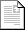ASEE Zone 2 Conference 2017

Full Program »

Fluid Mechanics Laboratory Experiment: Calibration of a Semi Circular Weir

 Final Paperpdf288KB

Experiments in open channel flow are important especially to Civil Engineering students. Flow rate is commonly measured in an open channel by using a weir. Weirs that are used in our fluid mechanics lab include a V-notch weir, a suppressed edge weir, and a contracted edge weir. Each of these weirs has been studied extensively, and calibration methods/results have been published in many undergraduate fluid mechanics texts. These weirs are modeled mathematically using an equation relating flow rate to liquid depth over the lowest point of the weir. In an effort to find an additional weir for study, the semi circular weir was proposed. Relating flow rate to liquid depth for this weir involves an infinite series. To make the semi circular weir useful in the lab would require the derivation of a single term, power law equation to relate flow rate to depth. The objective of this study is to derive such an equation, and to present data obtained for purposes of verification. Finding such an equation and avoiding an infinite series permits the use of the semi circular weir, as an additional weir experiment, in the fluid mechanics lab. The results indicate that the semi circular weir is a worthwhile addition to the study of weirs.

Author(s):

William Janna
Mechanical Engineering Department
University of Memphis
United States

Paul Palazolo
University of Memphis
United States

Jeffrey Marchetta
University of Memphis
United States